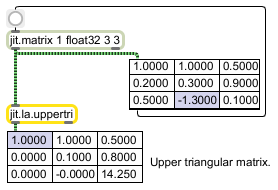jit.la.uppertri

Convert a matrix into an upper triangular matrix

Description

The jit.la.determinant object converts a given input matrix to an upper triangular matrix via Gaussian elimination. The input matrix must have typefloat32 or float64, and may have planecount 1 or 2. If the input matrix has a planecount of 2, it is assumed that the data is from the set of complex numbers.

Matrix Operator

matrix inputs:1, matrix outputs:1
 Name IOProc Planelink Typelink Dimlink Plane Dim Type out n/a 1 1 1 1 1 float32 float64

Attributes

Name Type g/s Description
swapcount int (get) The number of row swaps required to perform Gaussian elimination
thresh float The threshold value beneath which the absolute value of the result of internal calculations are considered to be equal to zero (default = 0.000000001)

Examples